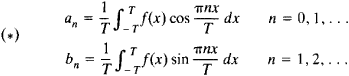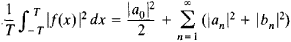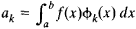# Fourier Coefficient

Also found in: Dictionary, Thesaurus.
Related to Fourier Coefficient: Fourier mode
The following article is from The Great Soviet Encyclopedia (1979). It might be outdated or ideologically biased.

## Fourier Coefficient

Fourier coefficients are the coefficientsin the Fourier series expansion of a periodic function f(x) with period 2Ƭ (see). Formulas (*) are sometimes called the Euler-Fourier formulas.

A continuous function f(x) is uniquely determined by its Fourier coefficients. The Fourier coefficients of an integrable function f(x) approach zero as n → ∞. Moreover, the rate of their decrease depends on the differentiability properties of f(x). For example, if f(x) has k continuous derivatives, then there is a number c such that |an| ≤ clnk and |bn| ≤ clnk. The Fourier coefficients are also connected with f(x) by the equality(seePARSEVAL EQUALITY). The Fourier coefficients of a function f(x) with respect to any normalized system of functions ϕ1(x), ϕ2, . . ., ϕn(x), . . . orthogonal on a segment [a, b] are given by the formula(seeORTHOGONAL SYSTEM OF FUNCTIONS).

References in periodicals archive ?
(4) Compute the Fourier coefficient matrix [mathematical expression not reproducible] by applying the 2D FFT.
To match the maximum order (330) of probed Fourier coefficient, the discretisation is set to [[DELTA].sub.f] = c/(2[DELTA]x(256/2 + 532)) [approximately equal to] 5.68 GHz which reflects the classical increment.
Continuous Current Waveforms with Nonpositive Fourier Coefficient of the Second (Third) Harmonic.
The following theorem gives the distribution of the Fourier coefficients and their relations under the general Levy-based model.
The values [{[??](S)}.sub.S[subset or equal to][n]] are often called the Fourier coefficients of f.
The following result describes the asymptotic behavior of the Fourier coefficient [[tau].sup.DtN.sub.mn] corresponding to the high-order spurious modes [u.sup.(4).sub.mn].
Consequently, Theorem 2.6 implies that the Fourier coefficients of Zf [bar.[Zg.sub.j]] belong to [l.sup.p.sub.m]([Z.sup.2d]) for each j = 1,..., 2d + 1.
The numerical method is facilitated by computational evidence establishing that with our choice of indices, and with the exception of some resonant cases, the Fourier coefficient [B.sub.mn] in the spectrum is largely determined by the corresponding coefficient [[DELTA].sub.mn] in the formula for the boundary of the plasma.
where ck are the Fourier coefficients of f defined by
where [c.sub.m]([[phi].sup.(0)]) is the Fourier coefficient of [[phi].sup.(0)].
Assume m lowest-frequency Fourier coefficients (frequency bins) are to be extracted; the frequency component extraction is achieved by converting [y.sub.ij] in (2) into 3):
The new cost function [??]([theta], [phi]) of every grid can be calculated efficiently by applying 2-D IFFT to the zeros padded Fourier coefficient matrix [MATHEMATICAL EXPRESSION NOT REPRODUCIBLE IN ASCII].

Site: Follow: Share:
Open / Close